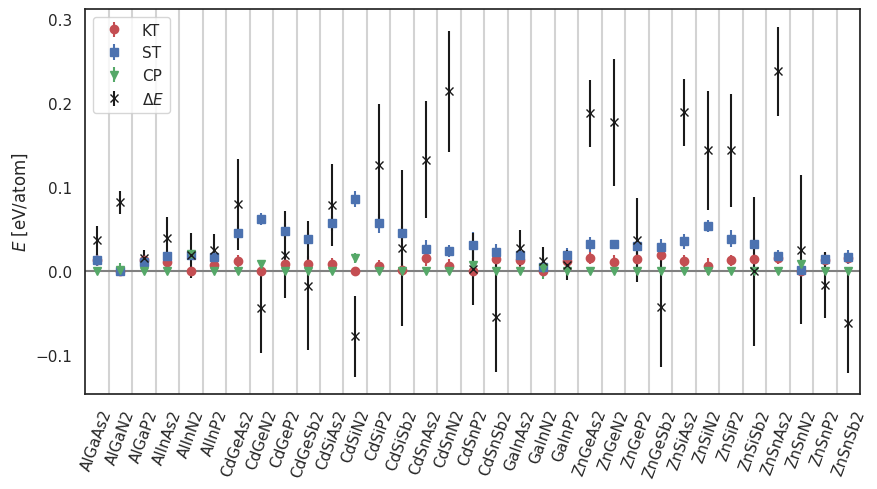# Database of ABX2 materials¶

Database contains chalcopyrite, kesterite, and wurtzite polymorphs of II–IV–V2 and III–III–V2 materials investigated for light absorption in the visible range. The data set includes stability information, band gaps, and carrier effective masses.

Mohnish Pandey, Korina Kuhar, and Karsten W. Jacobsen

II–IV–V2 and III–III–V2 Polytypes as Light Absorbers for Single Junction and Tandem Photovoltaic Devices

1. Phys. Chem. C, 2017, 121 (33), pp 17780–17786

## Key-value pairs¶

key

description

unit

Dxc

Derivative discontinuity (GLLB-SC)

eV

E_hull

Energy of the convex hull (with respect to the most stable structure)

eV

E_relative_perAtom

Energy per atom (with respect to the most stable structure)

eV

E_uncertanty_hull

Uncertanty of the convex hull energy

eV

E_uncertanty_perAtom

Uncertanty of the total energy

eV

GLLB_dir

Direct band gap (GLLB-SC)

eV

GLLB_ind

Indirect band gap (GLLB-SC)

eV

KS_gap

Kohn Sham band gap

eV

TB09_dir

Direct band gap (TB09)

eV

TB09_ind

Indirect band gap (TB09)

eV

lattice

Crystal system

m_e

Effective electron mass

m<sub>e</sub>

m_h

Effective hole mass

m<sub>e</sub>

phase

Phase: ZB=zinckblende, WZ=wurtzite, KT=wz kesterite, ST=wz stannite, CP=zb chaclopyrite

short_name

Short chemical formula

total_en

Total energy (mBEEF)

eV

## Example¶

mBEEF energies and the corresponding uncertainties for three phases:```# creates: enthalpy.png
import numpy as np
import matplotlib.pyplot as plt
from ase.db import connect

db = connect('abx2.db')
names = sorted(row.short_name for row in db.select(phase='ST'))
x = np.arange(len(names))

plt.figure(figsize=(10, 5))

for phase, mark, color in zip(['KT', 'ST', 'CP'], 'osv', 'rbg'):
y = []
dy = []
for row in db.select(phase=phase, sort='short_name'):
y.append(row.E_relative_perAtom)
dy.append(row.E_uncertanty_perAtom)
plt.errorbar(x, y, yerr=dy, color=color, fmt=mark, label=phase)

y = []
dy = []
for row in db.select(phase='ST', sort='short_name'):
y.append(row.E_hull)
dy.append(row.E_uncertanty_hull)
plt.errorbar(x, y, yerr=dy, color='k', fmt='x', label=r'\$\Delta E\$')
plt.xticks(x, names, rotation=70)
for xx in x:
plt.axvline(xx + 0.5, color='lightgrey')
plt.axhline(0, color='grey')
plt.xlim(-0.5, x[-1] + 0.5)
plt.legend()
plt.ylabel(r'\$E\$ [eV/atom]')
plt.savefig('enthalpy.png', bbox_inches='tight')
```